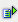The function time (iHour, iMinute, iSecond) calculates the time from the numerical values for hour (iHour), minute (iMinute) and second (iSecond).

The cell automatically gets the format string hh:mm:ss.

Syntax: =time(iHour,iMinute,iSecond)

Transfer parameters

Valid range

Comment

iHour

[0-23]

Values out of the valid range are set to the upper or lower limit

iMinute

[0-59]

Values out of the valid range are set to the upper or lower limit

iSecond

[0-59]

Values out of the valid range are set to the upper or lower limit

Result format: timeExample =time(12,24,48) is 12:24:48 Cell A1=12, cell A2=24, cell A3=48 Cell A4=time(A1,A2,A3) is 12:24:48